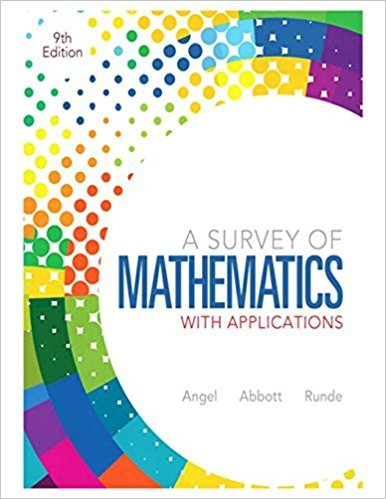×
×

# Solutions for Chapter 10: Mathematical Systems## Full solutions for A Survey of Mathematics with Applications | 9th Edition

ISBN: 9780321759665Solutions for Chapter 10: Mathematical Systems

Solutions for Chapter 10
4 5 0 389 Reviews
27
1
##### ISBN: 9780321759665

Chapter 10: Mathematical Systems includes 66 full step-by-step solutions. Since 66 problems in chapter 10: Mathematical Systems have been answered, more than 71110 students have viewed full step-by-step solutions from this chapter. This expansive textbook survival guide covers the following chapters and their solutions. This textbook survival guide was created for the textbook: A Survey of Mathematics with Applications, edition: 9. A Survey of Mathematics with Applications was written by and is associated to the ISBN: 9780321759665.

Key Math Terms and definitions covered in this textbook
• Complete solution x = x p + Xn to Ax = b.

(Particular x p) + (x n in nullspace).

• Complex conjugate

z = a - ib for any complex number z = a + ib. Then zz = Iz12.

• Diagonal matrix D.

dij = 0 if i #- j. Block-diagonal: zero outside square blocks Du.

• Fibonacci numbers

0,1,1,2,3,5, ... satisfy Fn = Fn-l + Fn- 2 = (A7 -A~)I()q -A2). Growth rate Al = (1 + .J5) 12 is the largest eigenvalue of the Fibonacci matrix [ } A].

• Hilbert matrix hilb(n).

Entries HU = 1/(i + j -1) = Jd X i- 1 xj-1dx. Positive definite but extremely small Amin and large condition number: H is ill-conditioned.

• Inverse matrix A-I.

Square matrix with A-I A = I and AA-l = I. No inverse if det A = 0 and rank(A) < n and Ax = 0 for a nonzero vector x. The inverses of AB and AT are B-1 A-I and (A-I)T. Cofactor formula (A-l)ij = Cji! detA.

• Linearly dependent VI, ... , Vn.

A combination other than all Ci = 0 gives L Ci Vi = O.

• Multiplication Ax

= Xl (column 1) + ... + xn(column n) = combination of columns.

• Multiplier eij.

The pivot row j is multiplied by eij and subtracted from row i to eliminate the i, j entry: eij = (entry to eliminate) / (jth pivot).

• Nullspace matrix N.

The columns of N are the n - r special solutions to As = O.

• Orthogonal subspaces.

Every v in V is orthogonal to every w in W.

• Polar decomposition A = Q H.

Orthogonal Q times positive (semi)definite H.

• Rayleigh quotient q (x) = X T Ax I x T x for symmetric A: Amin < q (x) < Amax.

Those extremes are reached at the eigenvectors x for Amin(A) and Amax(A).

• Reduced row echelon form R = rref(A).

Pivots = 1; zeros above and below pivots; the r nonzero rows of R give a basis for the row space of A.

• Saddle point of I(x}, ... ,xn ).

A point where the first derivatives of I are zero and the second derivative matrix (a2 II aXi ax j = Hessian matrix) is indefinite.

• Schwarz inequality

Iv·wl < IIvll IIwll.Then IvTAwl2 < (vT Av)(wT Aw) for pos def A.

• Symmetric matrix A.

The transpose is AT = A, and aU = a ji. A-I is also symmetric.

• Trace of A

= sum of diagonal entries = sum of eigenvalues of A. Tr AB = Tr BA.

• Triangle inequality II u + v II < II u II + II v II.

For matrix norms II A + B II < II A II + II B II·